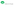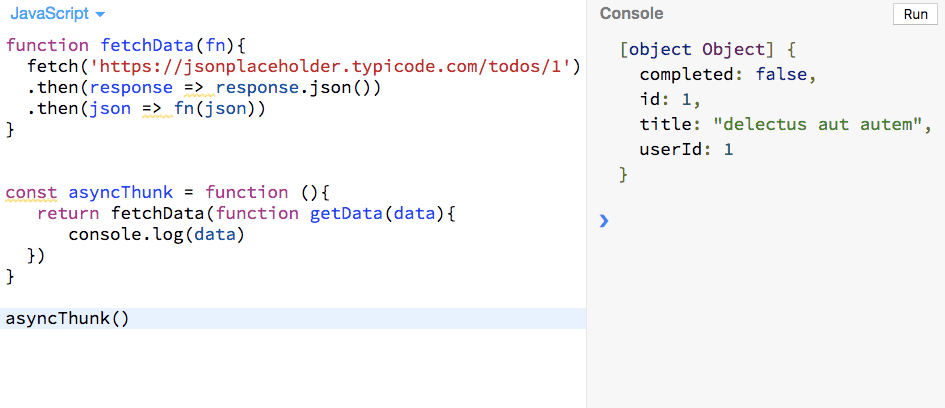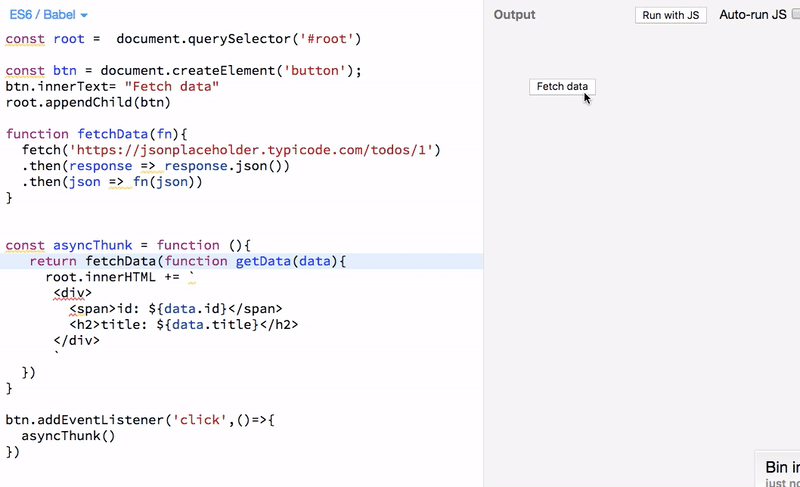# Intro to Thunks in JavaScriptIn this tutorial, we will learn about what are thunks and how to use the thunks in our JavaScript code.

### Definition of thunk

A thunk is just a function which delays the evaluation of the value.

The thunk function doesn’t take any arguments and gives the value whenever you invoke the thunk.

Example of synchronous thunk

``````function subtract(x,y){
return x-y;
}

// subtract function is evaluated the
// expression and result is returned immediately
console.log(subtract(5,3)) // 2

// this is a thunk function

// result is delayed untill we call the thunk() function
const thunk = function (){
return subtract(5,3);
}

// we always get the same data whenever we call the thunk function
console.log(thunk()) // 2``````

In the above code, we first defined the `subtract` function and next we defined `thunk` function which returns the value of `subtract` function.

### Asyncrouchronous thunks

Sometimes we need to make network requests in such case we need to use asynchronous thunks.

If you know about redux state management library which uses the asynchronous thunks to delay the actions to dispatch.

Let’s see an example:

``````function fetchData(fn){
setTimeout(()=>fn(1),2000)
}

const asyncThunk = function (){
return fetchData(function(data){
console.log(data)
})
}

asyncThunk()

// 1``````

In the above code, we used `setTimeout` function to make our code asynchronous.

### Network requests example using thunks

``````function fetchData(fn){
fetch('https://jsonplaceholder.typicode.com/todos/1')
.then(response => response.json())
.then(json => fn(json))
}

const asyncThunk = function (){
return fetchData(function getData(data){
console.log(data)
})
}

asyncThunk()``````

In the above code we are not calling the `getData` function immediately we only call the `getData` function whenever the `data` is available from the API endpoint.In our case, we just logged the data in the console if you want to render the data in the dom you can do it.

### Rendering data exampleIn the above example we used `FetchData` button to invoke the `asyncThunk()` function then we render the data in the dom.

## Top Udemy Courses##### JavaScript - The Complete Guide 2020 (Beginner + Advanced)
45,614 students enrolled
52 hours of video content
View Course##### React - The Complete Guide (incl Hooks, React Router, Redux)
284,472 students enrolled
40 hours of video content
View Course##### Vue - The Complete Guide (w/ Router, Vuex, Composition API)
130,921 students enrolled
48.5 hours of video content
View Course